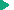SQL Reference
SQL Reference : OpenEdge SQL Language Elements : Data types : Bit string data types

Bit string data types
Bit string data types are used to define bit strings, which are sequences of bits having the value of either 0 or 1.

## Syntax

This is the syntax for a bit string data type:
 BIT | BINARY | VARBINARY | BLOB [ ( length ) ]

## Parameters

BIT
Corresponds to a single bit value of 0 or 1.
SQL statements can assign and compare values in BIT columns to and from columns of types CHAR, VARCHAR, BINARY, VARBINARY, TINYINT, SMALLINT, and INTEGER. However, in assignments from BINARY and VARBINARY, the value of the first four bits must be 0001 or 0000.
No arithmetic operations are allowed on BIT columns.
BINARY [ ( length ) ]
Corresponds to a bit field of the specified length of bytes. The default length is 1 byte. The maximum length is 2000 bytes.
When inserting literals into binary data types, INSERT statements must use a special format to store values in BINARY columns. They can specify the binary values as a bit string, hexadecimal string, or character string. INSERT statements must enclose binary values in single‑quote marks, preceded by b for a bit string and x for a hexadecimal string.
The following table lists the specification formats for binary values.
 Specification Format Example Bit string b '' b '1010110100010000' Hexadecimal string x '' x 'ad10' Character string '' 'ad10'
SQL interprets a character string as the character representation of a hexadecimal string.
If the data inserted into a BINARY column is less than the length specified, SQL pads it with zeros.
BINARY data can be assigned and compared to and from columns of type BIT, CHAR, and VARBINARY. Arithmetic operations are not allowed.
VARBINARY ( length )
Corresponds to a variable‑length bit field of the specified length in bytes. The default length is 1 byte. The maximum length is 31,995 bytes. The default length is 1. Due to index limitations, only the narrower VARBINARY columns can be indexed.
LVARBINARY/BLOB ( length )
Corresponds to an arbitrarily long byte array with the maximum length defined by the amount of available disk storage up to 1,073,741,823. A BLOB is an object of data type LVARBINARY.Maximum length for VARBINARYBLOB limitationsLanguage support for BLOBUtility support for BLOBCLOB limitationsUtility support for LVARCHAR (CLOB)